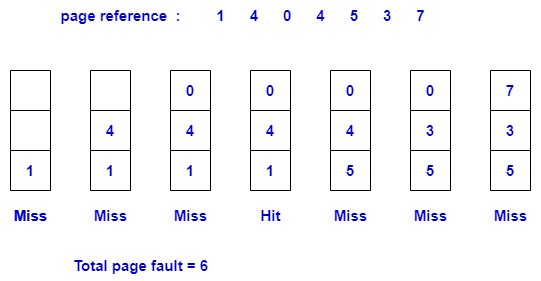# C program to implement the FIFO page-replacement algorithm

FIFO page-replacement algorithm is a technique in which a new page replaces the page which has been in the main memory for the longest time. This means the page which comes in the frame first will replace first.

In this algorithm, we will use a queue data structure.When the page number is added in a frame and the page number is not present in a frame then a page miss will occur and if the page number is present in a frame then a page hit occurs.

``````#include <stdlib.h>
#include<stdio.h>

int pagefault(int a[], int frame[], int n, int no) {
int i, j, avail, count = 0, k;
/* initialize frame with value -1 */
for (i = 0; i < no; i++) {
frame[i] = -1;
}
j = 0;
for (i = 0; i < n; i++) {
avail = 0;
for (k = 0; k < no; k++)
/* if equal it means page number(reference) is available in frame */
if (frame[k] == a[i])
avail = 1;
/* if avail=0 means page is not available in frame */
if (avail == 0) {
frame[j] = a[i];
/* j will calculate the position at which the new page add */
j = (j + 1) % no;
count++;  // variable count calculates the total page fault
}
}
return count;
}
void main() {
int n, i, * a, * frame, no, fault;
printf("\nENTER THE NUMBER OF PAGES:\n");
scanf("%d", & n);

a = (int * ) malloc(n * sizeof(int));
printf("ENTER THE PAGE NUMBER :\n");
for (i = 0; i < n; i++)
scanf("%d", & a[i]);

printf("ENTER THE NUMBER OF FRAMES :");
scanf("%d", & no);

frame = (int * ) malloc(no * sizeof(int));
fault = pagefault(a, frame, n, no);
printf("Page Fault Is %d", fault);
}
``````
output
```ENTER THE NUMBER OF PAGES:
7
ENTER THE PAGE NUMBER :
1 4 0 4 5 3 7
ENTER THE NUMBER OF FRAMES :3
Page Fault Is 6```